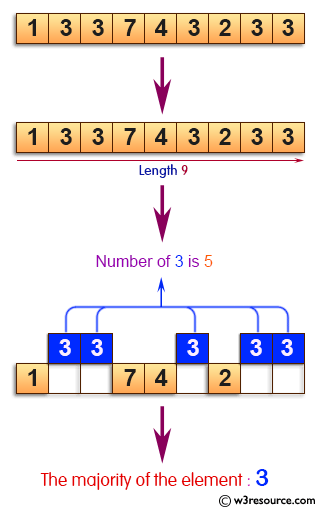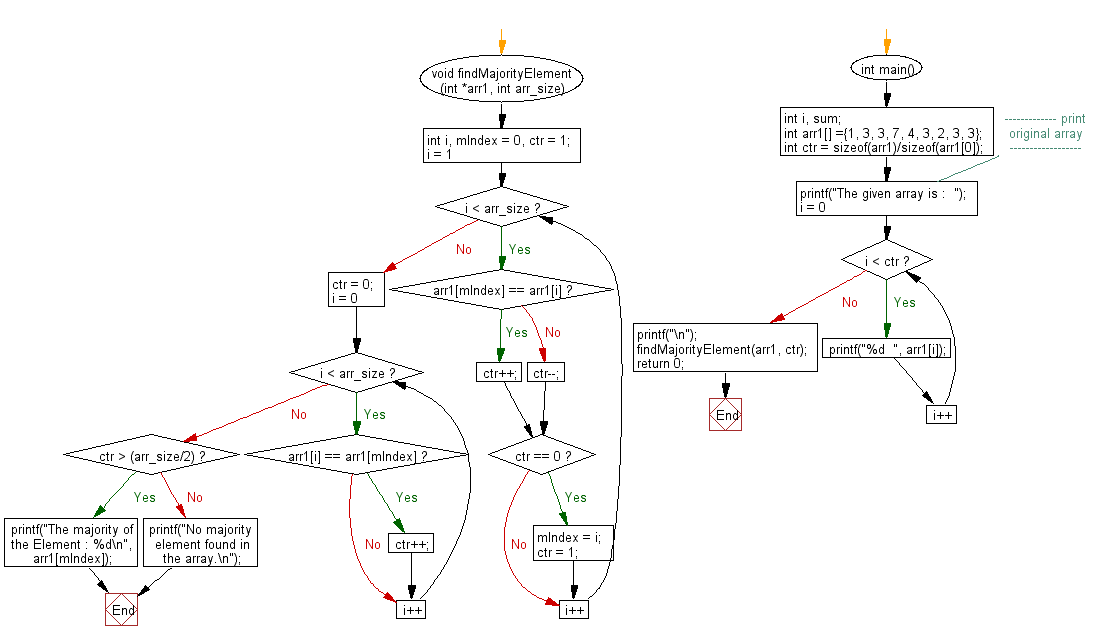﻿ C exercises: Find majority element of an array - w3resource# C Exercises: Find majority element of an array

## C Array: Exercise-49 with Solution

Write a program in C to find majority element of an array.

Pictorial Presentation:Sample Solution:

C Code:

``````# include <stdio.h>

void findMajorityElement(int *arr1, int arr_size)
{
int i, mIndex = 0, ctr = 1;
for(i = 1; i < arr_size; i++)
{
if(arr1[mIndex] == arr1[i])
ctr++;
else
ctr--;
if(ctr == 0)
{
mIndex = i;
ctr = 1;
}
}
ctr = 0;
for (i = 0; i < arr_size; i++)
{
if(arr1[i] == arr1[mIndex])
ctr++;
}
if(ctr > (arr_size/2))
printf("The majority of the Element : %d\n", arr1[mIndex]);
else
printf("No majority element found in the array.\n");
}
int main()
{
int i, sum;
int arr1[] ={1, 3, 3, 7, 4, 3, 2, 3, 3};
int ctr = sizeof(arr1)/sizeof(arr1);
//------------- print original array ------------------
printf("The given array is :  ");
for(i = 0; i < ctr; i++)
{
printf("%d  ", arr1[i]);
}
printf("\n");
//------------------------------------------------------
findMajorityElement(arr1, ctr);
return 0;
}```
```

Sample Output:

```The given array is :  1  3  3  7  4  3  2  3  3
The majority of the Element : 3
```

Flowchart:C Programming Code Editor:

Improve this sample solution and post your code through Disqus.

What is the difficulty level of this exercise?

﻿

## C Programming: Tips of the Day

Where is the C auto keyword used?

auto is a modifier like static. It defines the storage class of a variable. However, since the default for local variables is auto, you don't normally need to manually specify it.

Ref : https://bit.ly/3yzwC9r